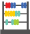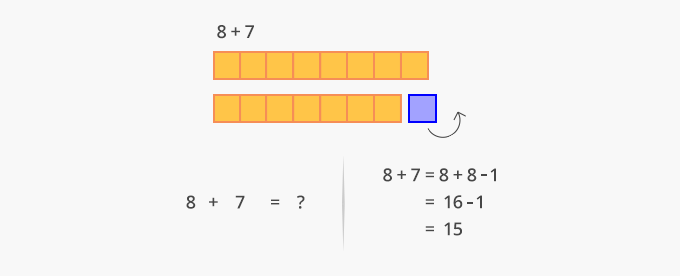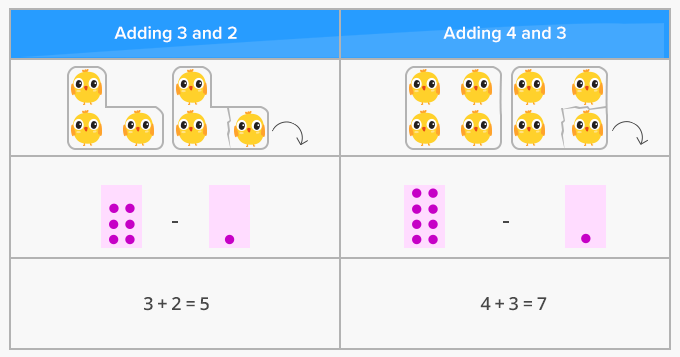# Doubles Minus 1 - Definition with Examples

The Complete K-5 Math Learning Program Built for Your Child

• 40 Million Kids

Loved by kids and parent worldwide

• 50,000 Schools

Trusted by teachers across schools

• Comprehensive Curriculum

Aligned to Common Core

##Let's learn!

What is doubles minus 1?
Doubles minus 1 is a strategy used to add two consecutive numbers that is, when they are next to each other.
We simply add the bigger number twice or double it and then, subtract 1 from it, to get the final result.

Here, for example, consecutive number 7 and 8 have been added using the doubles minus one strategy.Here are more examples of the double minus 1 strategy.Fun Facts Doubles minus 1 and doubles plus 1 are also called near doubles strategies.

##Let's sing!

Adding five and six; there’s no trouble!
Just make the bigger number double,
Subtract 1 from the double of bubbles.

##Let's do it!

Have fun with your child by asking them to find the doubles of numbers you say and then use double minus 1 and doubles plus 1 strategies to find the sum of consecutive numbers between 1 and 20.

##Related math vocabulary

Won Numerous Awards & Honors